# How to use the NUMBERVALUE function in Excel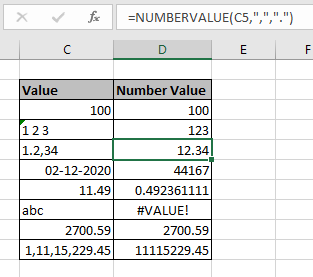In this article, we will learn about how to use the NUMBERVALUE function in Excel to extract number out of a given input text value using the specified decimal and group separators.

What is NUMBERVALUE function?

NUMBERVALUE function in excel returns a number in numerical value converted from any format using custom separators.

Syntax of NUMBERVALUE function:

=NUMBERVALUE ( text, [decimal_separator], [group_separators])

text : any value. It can text, number, date, time or currency.

decimal_separator : [optional] character which separates the integer part and fractional part. ( like 25.29)

group_separator : [Optional] character used to separate groupings of numbers in pairs of 3 ( like 100,000)

Example:

All of these might be confusing to understand. So, let's test this formula via running it on the example shown below. Here we have some random data values to test the NUMBERVALUE function in Excel.

Here we have some random values in different cell and we need to get the numerical value in number format using the below formula

Use the formula:

=NUMBERVALUE(C3)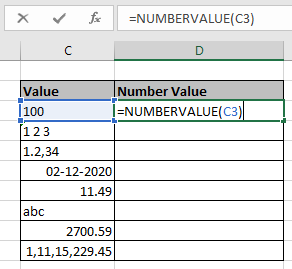Press Enter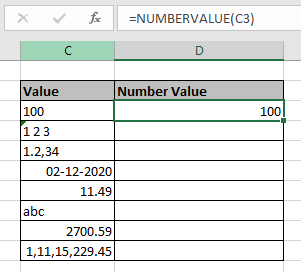Here 100 number value is returned from given text value. Now use the formula with separator for other cells like shown below.

Use the formula:

=NUMBERVALUE(C5,",",".")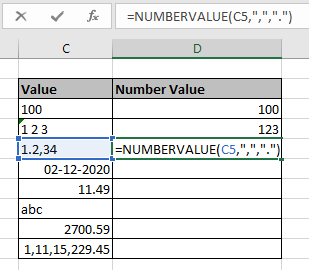See the formula in the cell. Here array value arguments to the function are given using the cell reference method.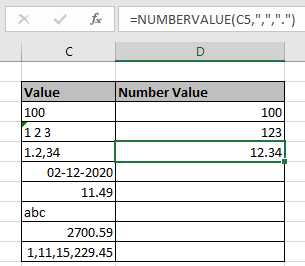Here the number 12.34 is obtained from the input value in C5. Now get the numerical value for other data values using the same formula.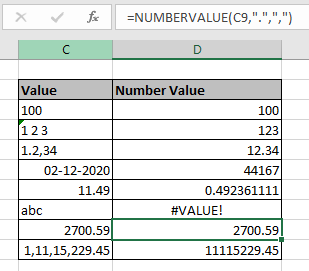Here are some observational notes shown below.

Notes:

1. Empty string as text in the function argument returns 0.
2. If the optional arguments decimal_separator and group _separator are not input, then current locale is used.
3. Empty spaces are ignored as in cell D4.
4. The formula returns #VALUE! Error :
1. If any of the arguments are not valid.
2. If the group separator occurs after the decimal separator.
3. If a decimal separator is used more than once in the Text argument.
5. The date, time or currency values also returns their numerical value.

Hope this article about How to use the NUMBERVALUE function in Excel is explanatory. Find more articles on number function formulas here. If you liked our blogs, share it with your fristarts on Facebook. And also you can follow us on Twitter and Facebook. We would love to hear from you, do let us know how we can improve, complement or innovate our work and make it better for you. Write to us at info@exceltip.com.

Related Articles

Convert month name to serial number in Excel : convert a column that holds the name of the month using DATEVALUE function in Excel

How to Use ISNUMBER Function in Excel : ISNUMBER function returns TRUE if number or FALSE if not in Excel.

How to Convert negative number to positive number in Excel : Absolute value means negative numbers are converted to positive and the positive numbers stay unaffected using ABS function in Excel.

Extract number between parentheses from text in Excel : Extract year value from the book name Argo(2012) using the SEARCH function in Excel.

Popular Articles:

50 Excel Shortcuts to Increase Your Productivity | Get faster at your task. These 50 shortcuts will make you work even faster on Excel.

The VLOOKUP Function in Excel | This is one of the most used and popular functions of excel that is used to lookup value from different ranges and sheets.

COUNTIF in Excel 2016 | Count values with conditions using this amazing function. You don't need to filter your data to count specific values. Countif function is essential to prepare your dashboard.

How to Use SUMIF Function in Excel | This is another dashboard essential function. This helps you sum up values on specific conditions.

1.Hi Dave,

Use Text to Column option.

Alt>A>E, step 1 click on Next, step 2 check to other box type decimal sign in box, then click on next, Step 3 click on Finish.

2.Hi,

How do I fix the decimal separator in Excel 2013. If I import files into Excel, it will not do calculations on it unless I retype the amount.

Terms and Conditions of use

The applications/code on this site are distributed as is and without warranties or liability. In no event shall the owner of the copyrights, or the authors of the applications/code be liable for any loss of profit, any problems or any damage resulting from the use or evaluation of the applications/code.